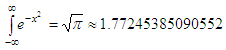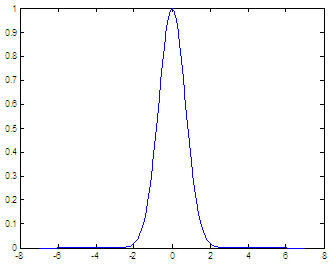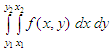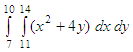Learn to solve definite integrals with a numerical software

How to calculate integrals with Matlab

 In computerized or numerical methods, a numerical integration can be performed by a number of algorithms that calculate the approximate value of definite integrals. Numerical evaluation of the integralis called quadrature. The term ‘numerical quadrature’ is more or less a synonym for numerical integration, especially if applied to one-dimensional integrals.

We’re going to focus this time on the calculation of definite integrals using the Matlab already built-in functions.

Why we can evaluate definite integrals

There’s a number of reasons for carrying out numerical integration. The integrand f(x) may be known only at certain points, such as obtained by sampling. Some digital systems and other computer applications may need integral calculus for this reason.

A formula for the integrand could be known, but it may be difficult or impossible to find an analytical integral.

It may be possible to find an antiderivative, but it may be easier to compute a numerical approximation. That may be the case if the exact integral is given as an infinite series, or if its evaluation requires a special function which is not available.

Matlab provides the following built-in functions for numerical integration:

Basic form: q = quad(FUN, A, B), which tries to approximate the integral of scalar-valued function FUN from A to B to within an error of 1 x 10-6 using recursive adaptive Simpson quadrature. The function Y = f(X) should accept a vector argument X and return a vector result Y, the integrand evaluated at each element of X.

Basic form: q = quadl(FUN, A, B), which tries to approximate the integral using high order recursive adaptive quadrature.

Let’s work on some examples and learn a little bit about intgral calculus...

Single integration

The Gaussian integral (aka Euler-Poisson integral or Poisson integral) is the integral of the Gaussian function over the entire real numbers.Since the result is exact for a known interval, let’s use this function as a guinea pig.

First, let’s plot it to see what we’re doing. We cannot go from -infinity to infinity, but we can use a more reasonable interval.

x = -7 : .1 : 7;
y = exp(-x.^2);
plot(x, y)

and we getNow, we see that if we integrate in the interval [-8, 8] we can get a very good answer. We don’t have to go beyond that.

You can define a separate function for the integrand, and use its name within the quad function. In this case, the expression is simple and we’re going to use it directly as an argument to the quadrature

format long
y1 = quad('exp(-x.^2)', -8, 8)
'exp(-x.^2)', -20, 20)

and the answers are

y1 = 1.77245489096641
y2 = 1.77245399494936

Obviously, the second answer is better than the first one (according to sqrt(pi)), due to the longer interval, but even the first answer is correct to 5 digits.

You can type help quad or help quadl on your Matlab command window to see a full description of the usage and examples of the functions.

Double integration

To evaluate definite integrals of the formthere’s the function dblquad. The basic syntax for double integration is:

II = dblquad(‘fxy_fun’, x_min, x_max, y_min, y_max)

Let’s compute the following double integralf = inline('x.^2 + 4*y');
ii = dblquad(f, 11, 14, 7, 10)

and the result is 1719

From 'Definite Integrals' to home

From 'Definite Integrals' to 'Calculus Problems'

 Trapezium Rule Simpson's Rule Derivatives Series and sequences Top